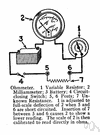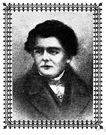# ohm

(redirected from Ohm (unit))
Also found in: Thesaurus, Medical, Financial, Encyclopedia.
Related to Ohm (unit): Ohm's law

## ohm

(ōm)
n.
The SI unit of electrical resistance, equal to the resistance of a conductor through which a current of one ampere flows given a one-volt potential across the conductor. See Table at measurement.

[After Georg Simon Ohm.]

## Ohm

(əʊm)
n
(Biography) Georg Simon (ˈɡeːɔrk ˈziːmɔn). 1787–1854, German physicist, who formulated the law named after him

## ohm

(əʊm)
n
(Units) the derived SI unit of electrical resistance; the resistance between two points on a conductor when a constant potential difference of 1 volt between them produces a current of 1 ampere. Symbol: Ω
[C19: named after Georg Simon Ohm ]
Collins English Dictionary – Complete and Unabridged, 12th Edition 2014 © HarperCollins Publishers 1991, 1994, 1998, 2000, 2003, 2006, 2007, 2009, 2011, 2014

## ohm

(oʊm)

n.
the SI unit of electrical resistance, equal to the resistance between two points when a constant potential difference applied between the points produces a current of 1 ampere.
[1861; after German. S. Ohm]

## Ohm

(oʊm)

n.
Georg Simon, 1787–1854, German physicist.

## ohm

(ōm)
A unit used to measure the electrical resistance of a material. One ohm is equal to the resistance of a conductor through which a current of one ampere flows when a potential difference of one volt is applied to it.

## ohm

1. The unit of electrical resistance.
2. (! ) A unit of electrical resistance. One ohm equals the resistance across which a potential difference of one volt produces a current flow of one ampere. Named after the German physicist G.S. Ohm (1787–1854).
Dictionary of Unfamiliar Words by Diagram Group Copyright © 2008 by Diagram Visual Information Limited
ThesaurusAntonymsRelated WordsSynonymsLegend:
 Noun 1ohm - a unit of electrical resistance equal to the resistance between two points on a conductor when a potential difference of one volt between them produces a current of one ampereresistance unit - the reciprocal of conductanceabohm - a unit of resistance equal to a billionth of an ohmmegohm - a unit of resistance equal to one million ohms 2Ohm - German physicist who formulated Ohm's law (1787-1854)Georg Simon Ohm
Based on WordNet 3.0, Farlex clipart collection. © 2003-2012 Princeton University, Farlex Inc.
Translations

## ohm

[əʊm] Nohmio m, ohm m
Collins Spanish Dictionary - Complete and Unabridged 8th Edition 2005 © William Collins Sons & Co. Ltd. 1971, 1988 © HarperCollins Publishers 1992, 1993, 1996, 1997, 2000, 2003, 2005

## ohm

nOhm nt
Collins German Dictionary – Complete and Unabridged 7th Edition 2005. © William Collins Sons & Co. Ltd. 1980 © HarperCollins Publishers 1991, 1997, 1999, 2004, 2005, 2007
Site: Follow: Share:
Open / Close